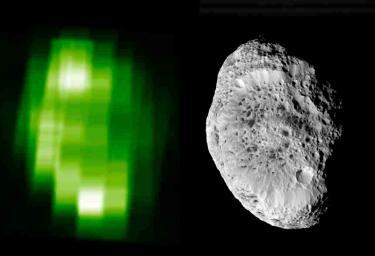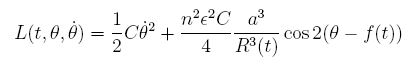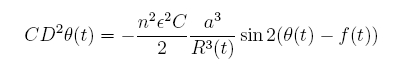On the Instability of Hyperion's Orbit

Michael Shaw December 10th, 2007

(Submitted as coursework for Physics 210, Stanford University, Fall 2007)Fig 1: Hyperion in ultraviolet, best suggesting the high and low albdeo regions, and in visible light. Note the large crater, and the assymetrical shape. (Courtesy of NASA/JPL-Caltech.)

Hyperion

Hyperion, the eigth known satellite of Saturn, was discovered in 1848 through an attempted observation of the satellite Iapetus. The observers saw an additional small moving dot on the sky, and over multiple days of observations, discovered it was also a moon orbiting Saturn.  Lassel was not the first to discover Hyperion--Bond discovered it two days earlier, but Lassell beat him to publication, both in Monthly Notices of the Royal Astronomical Society, a fairly new journal at the time. [1,2] Over the years, confusion over Hyperion was not centered on its disputed discovery, but on its unprecedentedly chaotic orbit.

Hyperion is sufficiently small that gravitational forces do not force it into a spherical shape. It is 360km along its longest axis, 280km along another, and 225km along the third.  This asymmetry in the axes will be a critical component of the discussion of chaos in section 3. Hyperion has a V magnitude of 14.2, visible to all but the smallest telescopes. It orbits Saturn once every 21.28 days, with an oribtal eccentricty of 0.1042, relatively large as far as solar system bodies go. Hyperion's surface is dominated by a few large craters, some a substantial fraction of the moon's size - one is 120km in length. 

Hyperion has a mass of .5584x1019kg, and therefore a mean density of .5667 g/cm3.  The low density suggests a composition high in ice, and low in rock (Hyperion, a moon of Saturn, is outside the ice line and it is reasonable to assume that it is formed partly of ice). Notwithstanding its icy composition, Hyperion has an albedo of 0.3, that is that only 30% of the sunlight hitting Hyperion is reflected. That albedo is relatively low, and suggets a darker surface than water ice. More precisely, in and around the rims of craters, Cassini's Ultraviolet Imaging Spectrometer (UVIS) finds regions of high albedo, suggesting the presence of relatively naked water ice. Spectral follow-up confirms the existence of H2O absorption features. The rest of the moon shows low albedo, weak H2O absorption, strong CO2 absorption, and presence of cyanides. This material is likely similar im composition to the crust of Phoebe, and one side of Iapetus. 

Of particular interest to this author is not the surface composition of Hyperion, but its orbital properties. We know that it orbits Saturn in a regular period, but nevertheless the orbit is chaotic. On the Earth, we have come to accept that one side of the planet will face the sun for (approximately) 12 hours, and then the other side will face the sun. On the moon, the aptly defined near side always faces the Earth. On Hyperion, we will show that the rotation about its own axis is chaotic on short time scales, and that therefore, the orientation of the planet cannot be analytically determined (without excessive effort).

Spin Orbit Coupled Lagrangian Mechanics

The toolbox we intend to use is that of Lagrangian mechanics. We treat the orbit of a satellite around a major planet (ie: Hyperion around Saturn) as a spin-orbit coupling. Throughout this discussion, we follow Sussman and Wisdom's canonical text. In particular, we consider the major planet to have a sufficiently large mass that perturbations in its orbit can be ignored. We develop the potential energy of the orbiting satellite by an integration over the satellite of its mass divided by the radius of that point to the central gravitational well. By expanding over Legendre polynomials, and considering only first and second order effects, we find the usual GMM'/R potential energy term and a second term GM'/2R3 multiplied by a sum of the moments of inertia: (A + B + C - 3I), where I is the moment of intertia of the entire staellite around the center of mass, and A,B, and C are the principal moments of inertia of the satellite. Qualitatively, this term represents the stronger gravitational pull on the fraction of the satellite nearer to the major planet. It is negligible for approximately spherical satellites, but pronounced for satellites as asymmetrical as Hyperion. 

We now know the potential energy term (or at least a sufficiently accurate approximation). The kinetic energy of such a satellite is the standard rigid body kinetic energy, explained in any classical mechanics text as the sum of the linear kinetic energy and the rotational kinetic energy (and usually expressed in terms of Euler angles). In particular, with no component of angular velocity except along the &theta axis, we find T = C&theta'2/2. We subtract the potential energy from the kinetic energy to find the Lagrangian, L = T - V:where &epsilon is the out of roundness parameter, derivable from the three principal moments of interia. The equations are confined to one degree of freedom (the coordinate &theta - f(t) ), and we can numerically integrate the equations as a function of time. We find the equations of motion:We interpret these equations of motion as setting equal the change in angular momentum and the applied gravity gradient torque. As long as the constants in front are relatively small, the equation can be considered as a perturbation of the motion. We see this situation for the Moon. We are accustomed to considering half of the moon as the near side, that which we can see. Nevertheless, multiple observations will yield slightly different near sides. Because the moon's orbit is slightly elliptical (eccentricity of 0.05), its orientation changes slightly over its orbital period. Nevertheless, numerical integrations of the equations of motion show another effect: if the moon is kicked by impact with a large asteroid, or impacted by some other force, its orientation begins to oscilate with a period of approximately 40 orbits, or three years. This free libration of the moon's orbit is a spin-orbit coupled effect, but one that induces periodic oscillation around a stable equilibrium. 

The Chaotic Orbit of Hyperion

The equations governing the Moon's orbit and that of Hyperion are mathematically identical. One might ask why we expect chaotic behavior from Hyperion when the Moon displays no such chaos--the scale of the perturbations is governed by the out of roundness--A + B + C - 3I. For the moon, this term is approximately four orders of magnitude smaller than for Hyperion--what constitutes a small perturbation of the Moon's orbit will be a major constituent of Hyperion's. 

The moon's orbital, like many satellites, is synchronous with its rotation. Most other satellites (such as the Earth) have relaxation periods so long that they retain their primordial spins. Hyperion, however, has a relaxation period of around 1 billion years and yet it is not in a synchronous orbit. While an argument could be made that it has not been in its current orbital configuration long enough to have relaxed to a synchronous orbit, the argument breaks down since Iapetus, with a similar relaxation time-scale, has relaxed to a synchronous orbit. The similar albedo and surface absorption features suggest the satellites have a common origin, and therefore have had similar opportunities to relax to a synchronous state.  Therefore, Hyperion should have so relaxed.

Integration of the equations of motion for Hyperion with various initial conditions suggests a curious trend. There is a large chaotic zone surrounding the synchronous resonance states in a surface of section plot. Poincare first hypothesized these dynamical instabilities a century ago, but he suggested that they would be too difficult to compute.  Modern computer technology allows us to numerically compute such chaos. Wisdom and Peale find that the chaotic zone emcompasses the 1:2, 1:1, and 2:1 synchronous resonances in the spin-orbit space, and that therefore, while Hyperion's rotation is no longer primordial, it cannot relax into a synchronous resonance with its orbit. It should instead be tumbling chaotically as its &theta - f moves through the chaotic space in the surface of section plot. 

Wisdom and Peale's model is appealing, in that it requires Hyperion's unique properties to ensure chaotic libration. Hyperion's large out of roundness and high eccentricity are both necessary. The out of roundness is relatievly unique for an object that size. The eccentricity is assured to continue over cosmic time by the 4:3 resonance Hyperion has with Titan. This stability of chaotic parameters is unique in the solar system.  Observations by Klavetter later verified this hypothesis. Over 4.5 orbital periods, Hyperion was seen to follow no periodic rotation state, but fits well to chaotic rotation.  We therefore accept the conclusion that Hyperion exhibits chaotic rotation, as predicted by Wisdom and Peale using Lagrangian mechanics and a numerical integrator. It is a striking triumph of classical mechanics.

References

 W. Lassell, "Discovery of a New Satellite of Saturn," Monthly Notices of the Royal Astronomical Society. 8, 9 (1848).

 W. C. Bond, "Discovery of a New Satellite of Saturn," Monthly Notices of the Royal Astronomical Society. 9, 1 (1848).

 P. Thomas and J. Veverka, "Hyperion: Analysis of Voyager Observations," Icarus 64, 414 (1985).

 R. A. Jacobson et al., "The Gravity Field of the Saturnian System from Satellite Observations and Spacecraft Tracking Data," Astrophys. J. 132, 2520 (2006).

 D. P. Cruikshank et al., "Surface Composition of Hyperion," Nature 448, 54 (2007).

 G. J. Sussmand and J. Wisdom, Structure and Interpretation of Classical Mechanics (MIT, 2001).

 J. Wisdom and S. J. Peale, "The Chaotic Rotation of Hyperion," Icarus 58 137 (1984).

 H. Poincare, Les Méthodes Nouvelle de la Mécanique Céleste (Gauthier-Villars, 1892).

 J. J. Klavetter, "Rotation of Hyperion. I. Observations," Astron. J. 97, 2 (1989).